Next: Exercises Up: Rigid Body Rotation Previous: Gyroscopic Precession

# Rotational Stability

Consider a rigid body for which all of the principal moments of inertia are distinct. Let. Suppose that the body is rotating freely about one of its principal axes. What happens when the body is slightly disturbed?

Let the body be initially rotating about the-axis, so that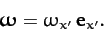(577)

If we apply a slight perturbation then the angular velocity becomes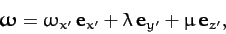(578)

where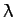andare both assumed to be small. Euler's equations (504)-(506) take the form(579)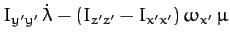(580)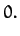(581)

Since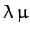is second-order in small quantities--and, therefore, negligible--the first of the above equations tells us that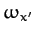is an approximate constant of the motion. The other two equations can be written(582)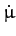(583)

Differentiating the first equation with respect to time, and then eliminating, we obtain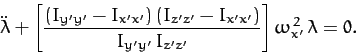(584)

It is easily demonstrated thatsatisfies the same differential equation. Since the term in square brackets in the above equation is positive, the equation takes the form of a simple harmonic equation, and, thus, has the bounded solution:(585)

Here,and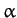are constants of integration, and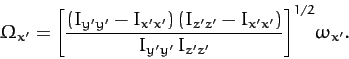(586)

Thus, the body oscillates sinusoidally about its initial state with the angular frequency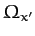. It follows that the body is stable to small perturbations when rotating about the-axis, in the sense that the amplitude of such perturbations does not grow in time.

Suppose that the body is initially rotating about the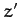-axis, and is subject to a small perturbation. A similar argument to the above allows us to conclude that the body oscillates sinusoidally about its initial state with angular frequency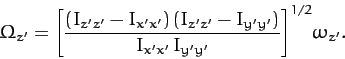(587)

Hence, the body is also stable to small perturbations when rotating about the-axis.

Suppose, finally, that the body is initially rotating about the-axis, and is subject to a small perturbation, such that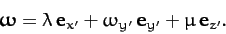(588)

It is easily demonstrated thatsatisfies the following differential equation: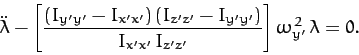(589)

Note that the term in square brackets is positive. Hence, the above equation is not the simple harmonic equation. Indeed its solution takes the form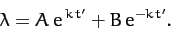(590)

Here,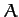andare constants of integration, and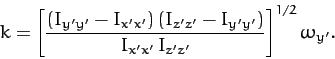(591)

In this case, the amplitude of the perturbation grows exponentially in time. Hence, the body is unstable to small perturbations when rotating about the-axis.

In conclusion, a rigid body with three distinct principal moments of inertia is stable to small perturbations when rotating about the principal axes with the largest and smallest moments, but is unstable when rotating about the axis with the intermediate moment.

Finally, if two of the principal moments are the same then it can be shown that the body is only stable to small perturbations when rotating about the principal axis whose moment is distinct from the other two.Next: Exercises Up: Rigid Body Rotation Previous: Gyroscopic Precession
Richard Fitzpatrick 2011-03-31